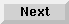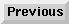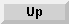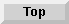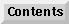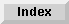5 Operators and Functions

## Operators and Expressions

An operator indicates a mathematical or logical operation to be performed on one or more values (operands) resulting in a single value. The combination of one or two operands with an operator is called an expression. For example, 10 + 5 is an expression consisting of two operands (10 and 5) and one operator (+).

The term operand can refer to a number, a string, or a variable.

An operator is generally placed between two operands, but some operators can precede a single operand. For instance, the minus sign is an operator which indicates subtraction when it appears between two operands (for example, 512-88), but it indicates negation when it appears before a single operand (for example, -1).

Some examples of expressions are as follows:

```
A - B
X + 1
"AB"&"CD"
-1
+2.14
2.14*
N*
N\$**
"STRING"**
```
* These are expressions in which the operator is assumed to be a plus sign (+).

** Strings or string variables can also be considered expressions.

Operators are divided into four classes depending on the kind of operation performed--arithmetic, string, relational, and logical (Boolean).

Eloquence Language Manual - 19 DEC 2002